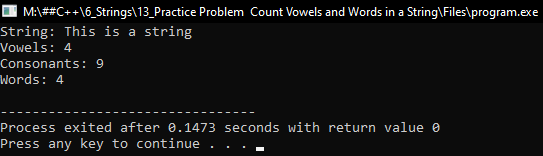# How to Count Vowels, Consonants and Words in a String in C++

## How to Count Vowels, Consonants, and Words in a String in C++

In this article, I am going to discuss How to Count Vowels, Consonants, and Words in a String in C++ Language with examples. Please read our previous article, where we discussed How to Change Cases of Letters of a String in C++ with examples.

##### How to Count Vowels, Consonants, and Words in a String in C++?

Here, we will write a program that will count vowels, consonants, and words in the given string. Let us see the program.

##### Program:
```#include <iostream>
#include <string.h>
using namespace std;
int main()
{
string str = "This is a string";
int vowels = 0, consonant = 0, space = 0;

for (int i = 0; str[i] != '\0'; i++)
{
if (str[i] == 'A' || str[i] == 'E' || str[i] == 'I' || str[i] == 'O'
|| str[i] == 'U' || str[i] == 'a' || str[i] == 'e' || str[i] == 'i'
|| str[i] == 'o' || str[i] == 'u')
vowels++;
else if (str[i] == ' ')
space++;
else
consonant++;
}

cout << "String: " << str << endl;
cout << "Vowels: " << vowels << endl;
cout << "Consonants: " << consonant << endl;
cout << "Words: " << space + 1 << endl;

return 0;
}
```
###### Output: